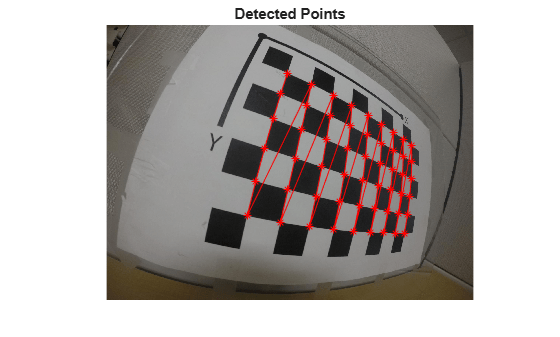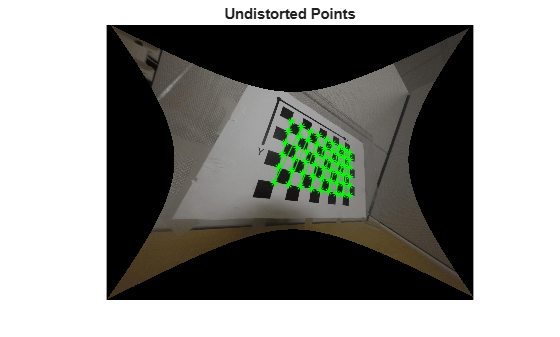# undistortFisheyePoints

Correct point coordinates for fisheye lens distortion

## Syntax

``undistortedPoints = undistortFisheyePoints(points,intrinsics)``
``undistortedPoints = undistortFisheyePoints(___,scaleFactor)``
``[___,camIntrinsics] = undistortFisheyePoints(___)``
``[___,reprojectionErrors] = undistortFisheyePoints(___)``

## Description

example

````undistortedPoints = undistortFisheyePoints(points,intrinsics)` returns point coordinates corrected for fisheye lens distortion.```
````undistortedPoints = undistortFisheyePoints(___,scaleFactor)` returns corrected point coordinates using the `scaleFactor` and the previous inputs. ```
````[___,camIntrinsics] = undistortFisheyePoints(___)` also returns a `cameraIntrinsics` object, which corresponds to a virtual perspective camera that produces undistorted points.```
````[___,reprojectionErrors] = undistortFisheyePoints(___)` also returns `reprojectionErrors` used to evaluate the accuracy of undistorted points. The function computes the reprojection errors by applying distortion to the points, and taking the distances between the result and the corresponding input points.```

## Examples

collapse all

Undistort and translate checkerboard points detected in a calibration image, and then display the results.

Create an `imageDatastore` object containing checkerboard calibration images.

```images = imageDatastore(fullfile(toolboxdir('vision'),'visiondata',... 'calibration','gopro')); imageFileNames = images.Files;```

Detect the calibration pattern from the images.

`[imagePoints,boardSize] = detectCheckerboardPoints(imageFileNames);`

Generate world coordinates for the corners of the checkerboard squares.

```squareSize = 29; % millimeters worldPoints = generateCheckerboardPoints(boardSize,squareSize);```

Estimate the fisheye parameters from the image and world points. Get the image size from the first image.

```I = readimage(images,10); imageSize = [size(I,1) size(I,2)]; params = estimateFisheyeParameters(imagePoints,worldPoints,imageSize);```

In the first image, detect the checkerboard points.

`points = detectCheckerboardPoints(I);`

Undistort the points and image.

```[undistortedPoints,intrinsics1] = undistortFisheyePoints(points,params.Intrinsics); [J, intrinsics2] = undistortFisheyeImage(I,params.Intrinsics,'OutputView','full');```

Translate the undistorted points.

```newOrigin = intrinsics2.PrincipalPoint - intrinsics1.PrincipalPoint; undistortedPoints = [undistortedPoints(:,1) + newOrigin(1), ... undistortedPoints(:,2) + newOrigin(2)];```

Display the results.

```figure imshow(I) hold on plot(points(:,1),points(:,2),'r*-') title('Detected Points') hold off``````figure imshow(J) hold on plot(undistortedPoints(:, 1),undistortedPoints(:, 2),'g*-') title('Undistorted Points') hold off```## Input Arguments

collapse all

Input points, specified as an M-by-2 matrix of M [x y] coordinates.

Fisheye intrinsic camera parameters, specified as a `fisheyeIntrinsics` object.

Scale factor for points, specified as a scalar or an ```[sx sy]``` vector. Specify a vector to scale the x and y axes individually. Increase the scale to zoom in the perspective of the camera view.

## Output Arguments

collapse all

Undistorted points, returned as an M-by-2 matrix of M number of [x y] coordinates. If `points` is `double`, then `undistortedPoints` is `double`. Otherwise, `undistortedPoints` is `single`.

Data Types: `single` | `double`

Undistorted intrinsics of a virtual camera, returned as a `cameraIntrinsics` object. These intrinsics are for a camera that has a perspective that produces the undistorted image.

Reprojection errors, returned as an M-by-1 vector. The function computes the reprojection errors by applying distortion to the undistorted points and taking the distances between the results and the corresponding input points. Errors are expressed in pixels.

## Version History

Introduced in R2017b